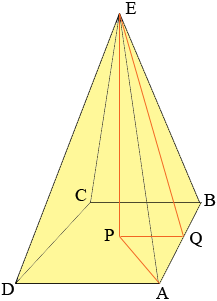SEARCH HOMEMath Central Quandaries & QueriesQuestion from Carla, a student: A symmetrical pyramid stands on a square base of side 8cm. The slant height of the pyramid is 20cm. Find the angle between the slant edge and the base, and the angle between a slant face and the base.Hi Carla,The angle between the slant face and the base is angle PQE. Triangle PQE is a right triangle, |QE| = 20 cm and |PQ| = 4 cm so cos(PQE) = 4/20 = 1/5. Hence the measure of angle PQE is cos-1(1/5).

A similar method can be used to find the measure of the angle PAE, the angle between the slant edge and the base by first applying Pythagoras theorem to triangles PAQ and EPA.

PennyMath Central is supported by the University of Regina and The Pacific Institute for the Mathematical Sciences.